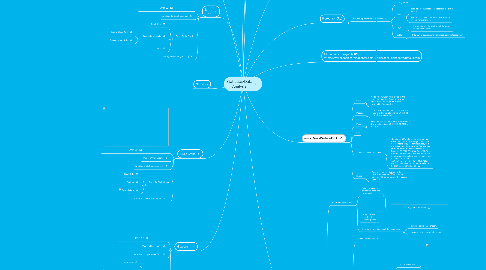# Statistics/Data Analysis

Get Started. It's FreeStatistics/Data Analysis## 1. Line Graph

### 1.5. What the graph needs?

1.5.1. includes the variable or quantity that changes

1.5.1.1. Units represent data

1.5.1.1.1. Main title for graph

## 2. Scatterplot

### 2.2. What graph needs?

2.2.1. Main title for graph

2.2.1.1. Descriptive label that includes the variable or quantity that changes

2.2.1.1.1. Units represent data

## 3. Bar Graph

### 3.3. Parts of a Bar Graph

3.3.1. Graph Title

3.3.2. Axes and their labels

3.3.2.1. Grouped Data Axis

3.3.2.2. Frequency Data Axis

3.3.3. Bars

## 4. Circle Graph

### 4.4. Parts of a Circle Graph

4.4.1. Graph Title

4.4.2. Sectors

4.4.3. Sector Labels

## 5. Histogram

### 5.4. Parts of a Histogram

5.4.1. Graph Title

5.4.2. Axes and their labels

5.4.2.1. Grouped Data Axis

5.4.2.2. Frequency Data Axis

5.4.3. Bars

5.4.3.1. Height

5.4.3.2. Width

## 6. Stem and Leaf Plot

### 6.2. What the graph needs?

6.2.1. Main title for the plot

6.2.1.1. complete number scale (stem) that accommodates the extreme values of the data set

6.2.1.1.1. Values for the "stem" written vertically from least (top) to greatest (bottom) values.

## 8. Measure of Central Tendency- KM,LR

### 8.1. Mean

8.1.1. Add all the numbers then divide by the number of numbers. Example- In a set of numbers {20, 22, 22, 23, 24} the mean is 22 after rounding.

### 8.2. Median

8.2.1. The middle number from least to greatest. Example- In a set of numbers {20, 22, 22, 23, 24} the median is 22.

### 8.3. Mode

8.3.1. Numbers that occur the most often. Example- In a set of numbers {20, 22, 22, 23, 24} the mode is 22.

### 8.5. Standard Deviation/Variance

8.5.1. The Standard Deviation is a measure of how spread out numbers are. Its symbol is σ (the Greek letter sigma) The formula is easy: it is the square root of the Variance. The average of the squared differences from the Mean. Example- 20,22,22,23,24/5 = Avg of 22.2 then subtract this with all the numbers and square it then divide this by how many numbers there is. It will look like this. 4.84+.04+.04+.04+.64+3.24=8.8/5 At last you find the square root to get the variance. So the final answer would be 1.32

### 8.6. Range

8.6.1. Biggest number subtracted by the smallest number. Example- In a set of numbers {20, 22, 22, 23, 24} the range is 24-20= 4

## 9. Pictograph (RL)

### 9.1. Used to represent tellies of categories.

9.1.1. Categorical data

9.1.2. Titles

9.1.2.1. Main title for the graph that describes the given data set.

9.1.2.2. Descriptive label that includes the variable or quantity that changes.

9.1.3. Legend

9.1.3.1. A key that gives the symbol and shows what the symbol represents.

9.1.4.1. http://www.superteacherworksheets.com/pictograph.html

## 10. Dot Plot (RL)

### 10.3. A horizontal number line on which each score is represented by a dot, or an x above the corresponding number-line value

10.3.1. Each x represents how many times an event occurred.

### 10.4. Outlier

10.4.1. A data point whose value is significantly greater than or less than other values.

### 10.5. Cluster

10.5.1. An isolated group of points.

### 10.6. Gap

10.6.1. A large space between data points.

### 10.7. Mode

10.7.1. Data value(s) that occur most often.

### 10.8. Bar Graphs

10.8.1. Shading in a dot plot in the squares of a grid paper and adding a vertical axis depicting the scale forms a bar graph.

## 12. Frequency Table (RL)

### 12.2. Characteristics

12.2.1. Each class interval has the same size.

12.2.2. The size of each interval can be computed by subtracting the lower endpoint from the higher and adding 1.

12.2.2.1.1. http://www.psychstat.missouristate.edu/introbook/sbk07.htm

12.2.3. The number of data values are known but the particular data values are unknown.

12.2.4. As the interval size increases, information is lost.

12.2.5. Classes (intervals) should not overlap.

## 13. Abuse of Statistics (KM, AG)

### 13.1. Reported Statistics-DG

13.1.1. Data interpretations are only as honest as their reporters

13.1.1.1. Registered for what?

13.1.2. Be objective and consider all evidence provided

13.1.3. Question information about the responders

13.1.3.1. How were participants chosen?

13.1.3.2. How were the responses interpreted?

13.2.1. Will be missing information

13.2.2. Check graphs for essential elements

13.2.2.1. Does it have a title?

13.2.2.1.1. There is no title on this graph.

13.2.2.2. Are there labels on the axes?

13.2.2.3. What is the source of the data?

13.2.2.4. Is there a key to a pictograph?

13.2.2.4.1. This graph is missing a key.

13.2.2.5. Does the pictograph use a uniform size of symbols?

13.2.2.6.1. What are we looking at? There is a key, but no title, no axis labels.

13.2.2.7. Are the numbers on the scale equally spaced?

### 13.3. Misuse of Mean, Median, and Mode

13.3.1. All of these figures are considered "averages"

13.3.2. These figures can be reported to suit the statistician's needs

13.3.3. Watch for a low number of contributors, which would allow an extremely high or low value skew the overall data